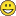# QlikView App Development

Discussion Board for collaboration related to QlikView App Development.

Highlighted
New Contributor II

## Qlik Sense - IF inside AVG or SUM

Hi everyone, today i tried to calculate an AVG for a metric like this:

```Avg(DISTINCT [Price])
```

But after that the user send me change, "We need calculate the price based in type of client, if client is type B the metric is Price * 1.21 and if the client isn´t B the metric is Price directly"

If i write separedly:

```Avg({<[Client_Type]={'B'}>}DISTINCT [Price]*1.21)
```

```Avg({<[Client_Type]=-{'B'}>}DISTINCT [Price])
```

The expressions working correctly, but i cant find a way to calculate this average completly.

I tried with:

```(Avg({<[Client_Type]={'B'}>}DISTINCT [Price]*1.21)+Avg({<[Client_Type]=-{'B'}>}DISTINCT [Price]))/2
```

But this expression shows a wrong value.

Is possible put a condicional calculation based in a dimension , inside a AVG or SUM  to show in a metric ?

Thanks in advanced1 Solution

Accepted SolutionsMVP

## Re: Qlik Sense - IF inside AVG or SUM

This is one option

Avg(DISTINCT If(Client_Type = 'B', [Price] * 1.21, [Price]))

2 RepliesMVP

## Re: Qlik Sense - IF inside AVG or SUM

This is one option

Avg(DISTINCT If(Client_Type = 'B', [Price] * 1.21, [Price]))

New Contributor II

Really Thanks